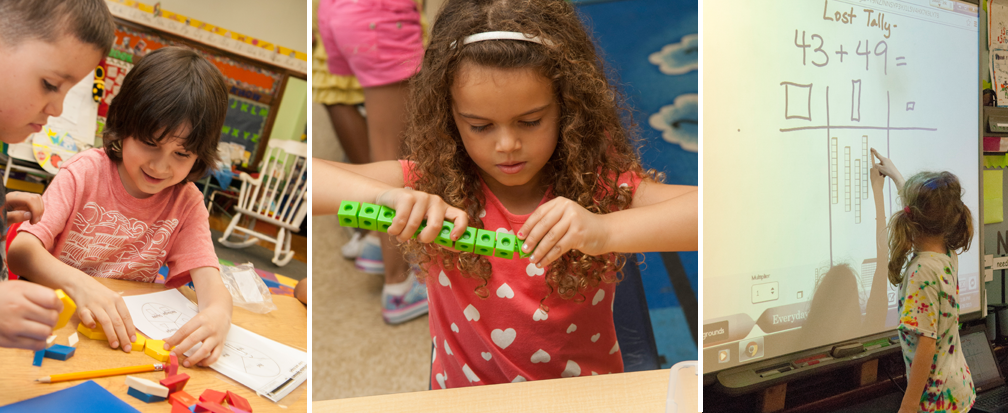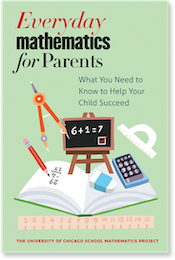## Basic Math Facts

### How does Everyday Mathematics address basic facts? When should students be expected to know their facts?

Helping children learn the basic facts is an important goal in the Everyday Mathematics curriculum. Most children should have developed an automatic recall of the basic addition and subtraction facts by the end of the second grade. They should also know most of their 1, 2, 5, and 10 multiplication facts by this time. By the end of the fourth grade most students should have an automatic recall of all the basic multiplication facts and be familiar with the basic division facts. Multiplication and division facts are reinforced at the beginning of fifth grade.

The Everyday Mathematics curriculum employs a variety of techniques to help children develop their "fact power", or basic number-fact reflexes. These include:

### Practice Through Games

The curriculum has a wide variety of fact practice games. Because children find these games much more engaging than standard drill exercises, they are willing and eager to spend more time practicing their basic facts.

For more information and instructions for playing sample games, visit our section on The role of Games in EM.

### Fact Triangles and Fact Families

Fact Triangles are Everyday Mathematics' flash cards with a difference. The difference is fact triangles help children learn fact families rather than isolated facts. Partner practice with addition and subtraction fact triangles begins in first grade. Multiplication and division fact triangles are introduced in second grade. Practice with Fact Triangles is often suggested in the Home Links homework assignments.

### 50-Facts Multiplication Tests

Beginning in fourth grade, students take timed tests on multiplication facts. These are recommended at least once every three weeks. Students calculate their percentage correct and track their progress with line graphs.

### Choral Drills and Mental Math Exercises

Beginning in first grade, short oral drills are suggested for fact review. These drills can become a routine during transitional times of the day. In fourth through sixth grades, basic fact power is reinforced in routine mental math exercises.

### Math Boxes

Math Boxes, which are sets of practice and review problems, are included with almost every lesson.

### Fact Extension Practice

Fact extensions are calculations with larger numbers by using knowledge of basic facts. If children know that 3 + 4 = 7, then they also know that 30 + 40 = 70, and 300 + 400 = 700. Children are introduced to fact extensions in first grade and are encouraged to practice them throughout the program.

### Frames and Arrows Diagrams

These diagrams are visual representations of rule-based sequences of numbers. Variations of these diagrams are used routinely from kindergarten through third grade. The challenge of solving these number puzzles by filling in the blank frames involves lots of practice with basic facts.

### “What's My Rule?” Function Machines

What's My Rule Variations of these function machines are used routinely through third grade, and provide another avenue for basic fact practice.

### Home Links

Home Link homework assignments are included with every lesson and have many opportunities for basic fact practice built into the suggested activities.Everyday Mathematics for Parents: What You Need to Know to Help Your Child Succeed

The University of Chicago School Mathematics Project

University of Chicago Press

Learn more >>

## Related Links

### Everyday Mathematics Online

With a login provided by your child's teacher, access resources to help your child with homework or brush up on your math skills.

### Understanding Everyday Mathematics for Parents

Learn more about the EM curriculum and how to assist your child.